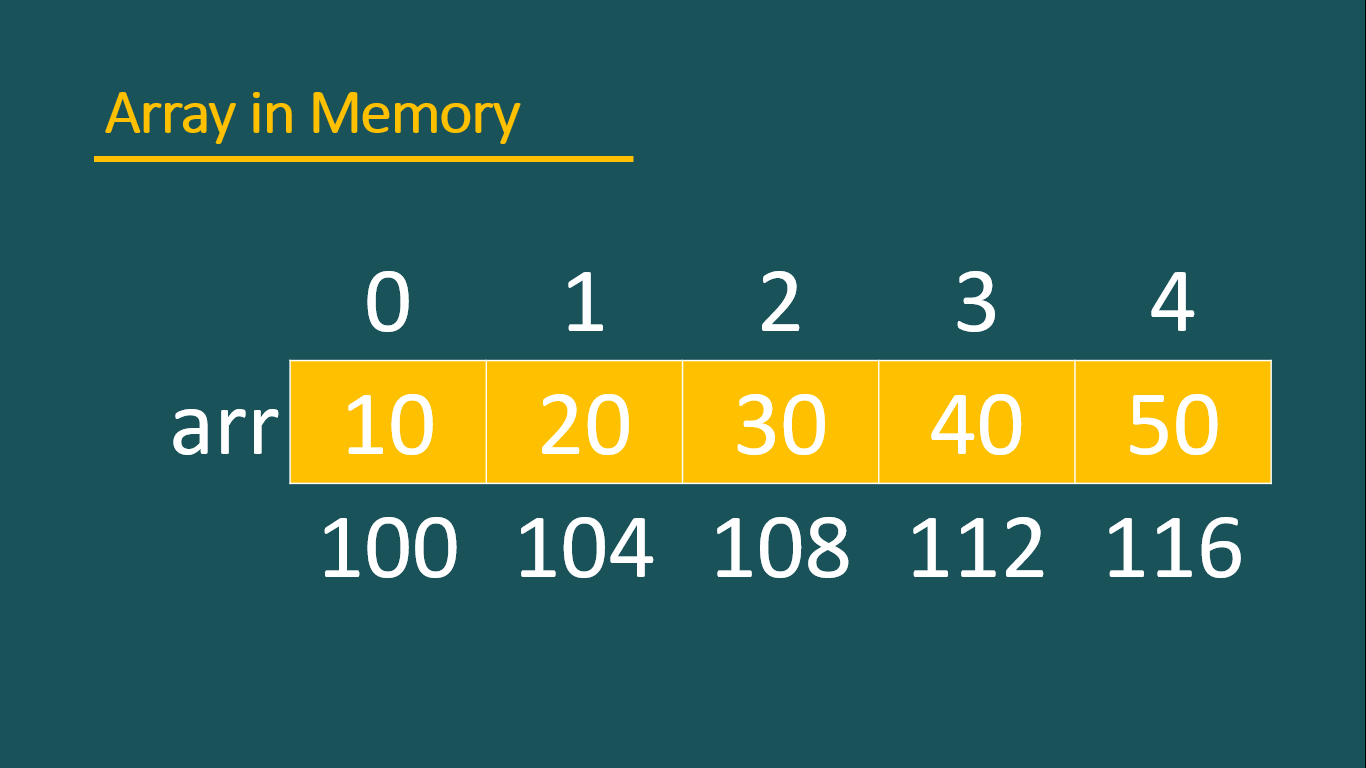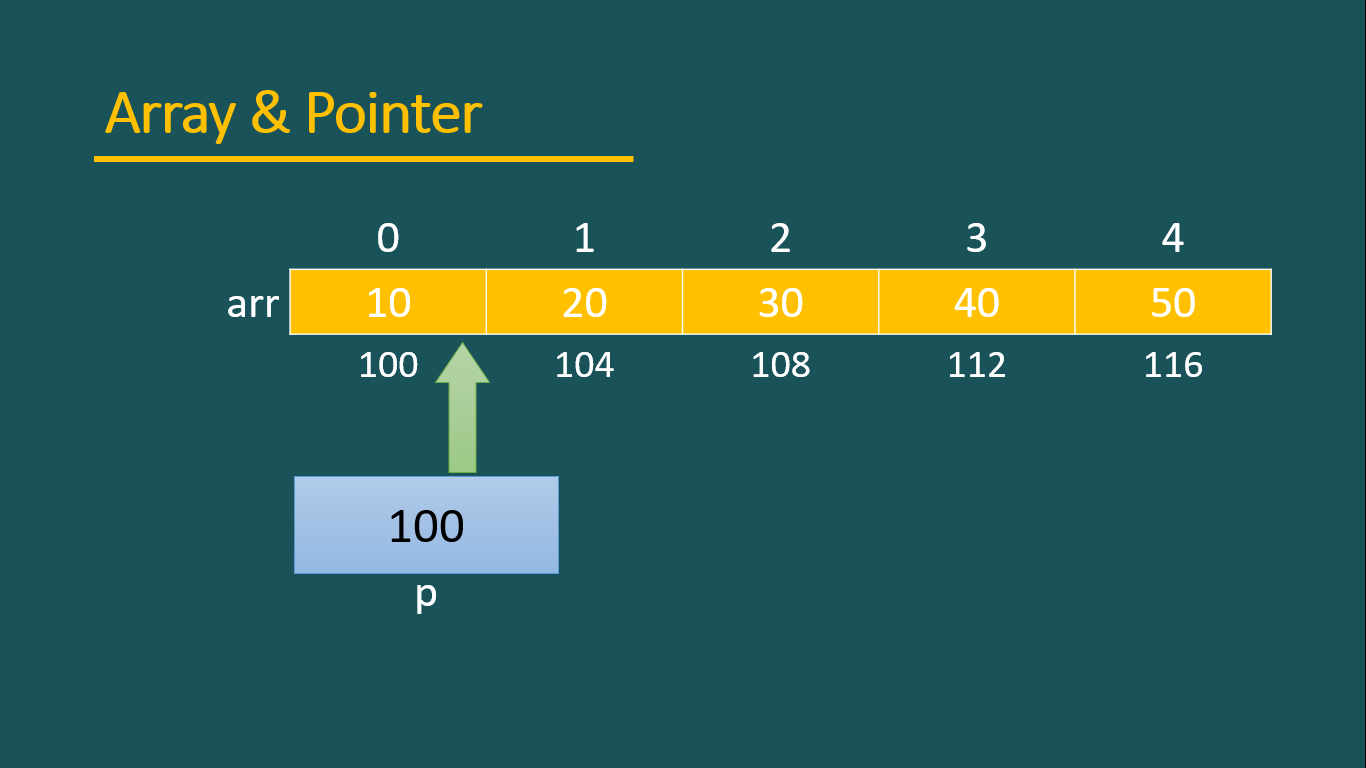# Explore hunderds of solved example topic wise.

## Arrays and pointer

Array and pointer are closely related to each other. In this article, we will discuss the relationship between array and pointer and learn how to access array elements using pointers also.

To understand the array and pointer relationship suppose we create an array which memory map is shown by the following figure. All the examples in this article use this memory map.```int arr[] = {10,20,30,40,50};
printf("%u\n",arr); //prints 100
printf("%u",&arr); //prints 100
```

Output

As shown in the above code snippet arr and &arr both print the same result and the result is the address of the first element of an array. The first element address of the array is also known as the base address of the array.

Note that to print the address %u format specifier is used because the address is always unsigned as we have discussed in the previous article arrays in c.

As shown in the above figure base address of an array is 100, it can vary if you run the above example on your computer.

### Storing array address into pointer

```int arr[] = {10,20,30,40,50};
int *p;
p = arr; //storing base address of array into pointer
```

As shown in the above code snippet array base address is stored into a pointer p. pointer p should be declared as an int* because it points the address of integer type array.

### Accessing array elements

```int arr[] = {10,20,30,40,50};
int *p;
p = arr; //storing base address of array into pointer

printf("%u\n",arr); //prints 100
printf("%u\n",p); //prints 100
printf("%u\n",*p); //prints 10
```
• As array name contains the base address of the array, so arr prints the base address.
• As pointer p store the address of the base address of array so the value of pointer p is the same as a base address.
• To access the base value (First element of the array) print the value at p by using *p.

### Pointer arithemitic

As we have discussed the pointer holds the address and the address is an unsigned integer. so arithmetic with pointers is also possible.

In pointers, arithmetic only ++,--,+,-,+= and -= operators are allowed with pointers means you can only perform addition and subtraction with pointers.```int arr[] = {10,20,30,40,50};
int *p = arr;

printf("%d",*p);//10
p++; //moves the pointer to the next element
printf("%d",*p);//20
p++;
printf("%d",*p);//30
p--;
printf("%d",*p);//20
```
1. As shown in the above example, initially pointer points the base address of the array.
2. p++ moves the pointer to the next element.
3. Similarly, p-- moves the pointer to the previous element.
4. As initially, p points the base address of the array, suppose the base address of the array is 100. After that p++ don't increase the value of p by 1 and make it 101. This happens because a normal variable increase by value whereas pointer increase by element means whenever a pointer is incremented it increase to point the next element.
5. So if the pointer points the integer array then each time pointer increments move the pointer 4 bytes and if the pointer points to the character array then pointer increment moves the pointer 1 byte ahead.

#### Addition and Subtraction to pointer

It is not necessary that a pointer is always increased by 1, to update pointer value more than 1 element by using += and -= operator as shown in the above example.

```int arr[] = {10,20,30,40,50};
int *p = arr;

printf("%d",*p); //10
p += 3; //shift pointer 3 elements ahead
printf("%d",*p); // 40;

p -= 2; //shift pointer 2 elements back.
printf("%d",*p);// 20;```

It is also desired that we actually don't want to shift the pointer, just wish to see the next elements without updating the pointer address. The following code snippet shows how to do this with addition(+) and subtraction(-) operator.

```int arr[] = {10,20,30,40,50};
int *p = arr; //storing the base address of array

printf("%d",*p); //10
printf("%d",*(p + 2)); //30
printf("%d",*p); //10

p += 2; // shift the pointer to the 3rd element
printf("%d",*p); //30
printf("%d",*(p + 1)); //40
```
• As shown in the above code snippet, pointer p initially holds the base address and when dereference prints the base value.
• In the next line, *(p + 2) access the element that is 2 elements ahead from the pointer current position but it actually doesn't shift the pointer. so in the next line *p again prints the base value.
• As shown in the above example, after shifting the pointer to 3rd element, *(p + 1) access the 4th element because the pointer is a relative reference and perform the addition and subtraction in the reference where pointer currently stands.

### Relation b/w array and pointer

An array is similar to a pointer actually array is a constant pointer. The following code snippet shows the relation between array and pointer.

arr[i] = *(arr + i) = *(i + arr) = i[arr];
The following code snippet demonstrates the above fact.
```int arr[] = {10,20,30,40,50};

printf("%d",arr); //30
printf("%d",*(arr + 2)); //30
printf("%d",*(2 + arr)); //30
printf("%d",2[arr]); //30
```

So from the above code snippet, it is clear that array also behaves like pointer and internally C programming treats the array as a pointer.

#### Why array name return base address

```int arr[] = {10,20,30,40,50};
printf("%d",arr); // 10
printf("%d",*(arr + 0)); //10
printf("%d",*arr); //10, base value
```
• As shown in the above code arr and *(arr + 0) are same and access the first element of the array.
• Similarly, *(arr + 0) and *arr are the same because the addition of 0 doesn't affect the array and print the first element.
• *arr print the base element (first element) so it is clear arr represent the base address.

#### Address calculation of array elements

1. As we have already learned array name (arr) represents the base address and (arr + 2) represents the address of 3rd-element of the array.
2. Actually, when we write (arr + 2) it internally converts like (arr + 2 * size_of_each_element). For example if arr is an integer array then the size of each element is 4 and if the base address of arr is 100 then the address of arr is calculated as given below...

(arr + 2) => (arr + 2 * 4) => (100 + 2 * 4) => 108. that is the address of 3rd element.

#### Difference between array and pointer

```int arr[] = {10,20,30,40,50};
int *p = arr;

printf("%d",*arr);//10;
printf("%d",*p);

p++;
printf("%d",*p); //20

arr++; //error,because array is a constant pointer
printf("%d",*arr);
```
As shown in the above code snippet, arr++ produces an error because an array is a constant pointer which address can't be updated and stick to the first element.